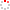#CodeStepByStep## mergeDigitPairs

Language/Type: C++ recursion

Write a recursive function named `mergeDigitPairs` that accepts an integer parameter n and returns the integer formed by combining each pair of digits from n into a single digit that is their sum. For example, if passed the number 1234, you should combine the digits 12 into 1+2 or 3, and combine the digits 34 into 3+4 or 7, leading to a returned result of 37.

If adding a given pair of digits produces a two-digit number, repeat the process until you have a single-digit number to replace the original pair. For example, if passed the number `1168`, the 11 becomes 1+1 or 2, but the 68 becomes 6+8 or 14, so we merge them again by saying that 14 is 1+4 or 5, so the pair 68 turns into 5, leading to an overall result of 25.

If passed a number with an odd number of digits, the first (most significant) digit is left untouched. For example, the number `13372` becomes `169` because the 3+3 becomes 6 and the 7+2 becomes 9. If passed a negative number, perform the same process as usual but return a negative result. For example, when passed `-1234`, return -37. If passed a single-digit number, simply return that number itself.

The following table shows several calls and their expected return values:

Call Return Value
`mergeDigitPairs(1234)` `37`
`mergeDigitPairs(3186507)` `3927`
`mergeDigitPairs(-52874)` `-512`
`mergeDigitPairs(88888888)` `7777`
`mergeDigitPairs(20581974)` `2412`
`mergeDigitPairs(0)` `0`
`mergeDigitPairs(6)` `6`
`mergeDigitPairs(6)` `-5`

Constraints: Do not declare any global variables. Also, do not use any loops; you must use recursion. Do not use a string to solve this problem. (For example, do not convert n into a string.) You are allowed to define other "helper" functions if you like; they are subject to these same constraints.

Type your C++ solution code here:

This is a function exercise. Write a C++ function as described. Do not write a complete program; just the function(s) above.